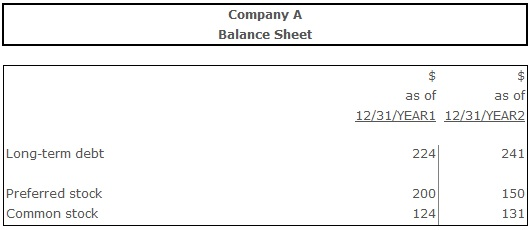# Long-Term Debt to Equity

Long-term Debt to Equity – an indicator of the creditors’ protection level in case of the firm’s insolvency, comparing its long-term debt with shareholders’ equity.

## Formula(s):

Long-Term Debt to Equity = The Long-Term Debt ÷ (Preferred Equity + Common Equity)

## Example:Long-Term Debt to Equity Ratio (Year 1) = 224 ÷ (200 + 124) = 0,69

Long-Term Debt to Equity Ratio (Year 2) = 241 ÷ (150 +131) = 0,85

## Conclusion:

In year 1 the long-term debt to equity ratio was 0,69. Year 2 witnessed the increase of this ratio to 0,85, indicating the decrease of creditors’ protection level in case of insolvency of a firm.